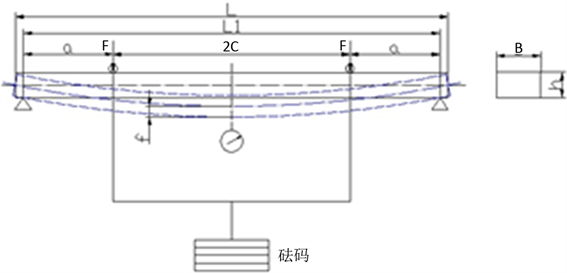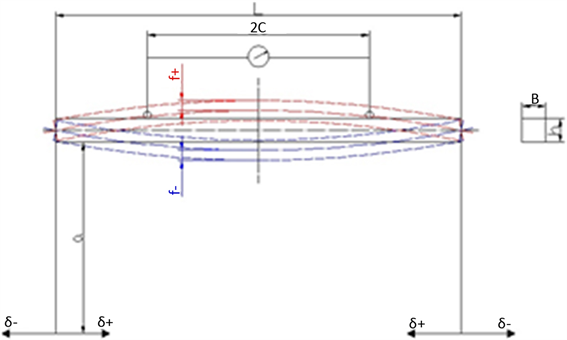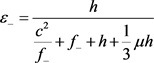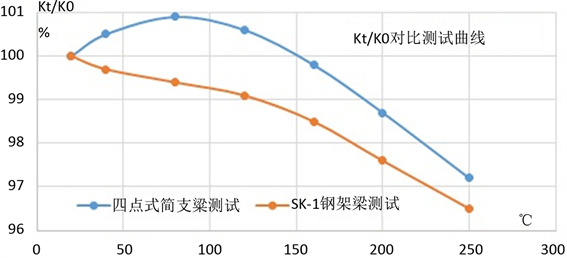﻿ 应变计灵敏系数标定影响因素分析

# 应变计灵敏系数标定影响因素分析Influence Factor Analysis for Strain Gage’s Gage Factor Calibration

Abstract: The accuracy of gage factor test affects the design and output of strain sensor as well as the au-thenticity of strain correction data in strain test. After the gage factor measuring model is analyzed which is based weights loading four points simply supported beam and pure bending steel frame beam structure, the influencing factors of standard strain will be confirmed. The influencing factors of gage factor calibration include the test beam size and the axial force of test beam. The test results of practical gage factor show that the factor of simple beam is slightly larger and the dispersion is obviously larger. The output of strain gage at temperature is related to the load strain, the size change of test beam, and the change of elastic modulus. Theoretically, the dimensional change and elastic modulus change of the simply supported beam at temperature can be caused error as high as 2.9%. The temperature influence error is very small, because the steel beam is the rigid closed static mechanical balance system. Using test, the theoretical analysis is verified by measuring the gage factor change with temperature which uses the simply supported beam and steel beam.

1. 引言

2. 应变计工作原理

$R=\rho \frac{nl}{ns}=\rho \frac{L}{S}$ (1)

$\frac{\Delta R}{R}=\frac{\Delta L}{L}-\frac{\Delta S}{S}+\frac{\Delta \rho }{\rho }$ (2)

$\frac{\Delta L}{L}=\epsilon$

$\frac{\Delta S}{S}=\frac{2\Delta r}{r}=-2\mu \frac{\Delta L}{L}=-2\mu \epsilon$

$\frac{\Delta R}{R}=\left(1+2\mu \right)\epsilon +\frac{\Delta \rho }{\rho }$ (3)

$K=\frac{\Delta R/R}{\epsilon }=1+2\mu +\frac{\Delta \rho /\rho }{\epsilon }$ (4)

3. 应变计灵敏系数测量模型原理

3.1. 砝码加载四点式简支梁结构$M=Fx-\frac{G}{2}\left(x-a\right)=\frac{G}{2}a$ (5)

$\epsilon =\frac{\delta }{E}=\frac{\frac{M}{W}}{E}=\frac{6Fa}{EB{h}^{2}}=\frac{3Ga}{EB{h}^{2}}$ (6)

 (7)

$\epsilon =\frac{12h}{3{L}_{1}^{2}-4{a}^{3}}×f$ (8)

3.2. 纯弯矩钢架梁结构Figure 2. Diagram of forces on pure moment steel frame beam

$M=\sigma a$ (9)

${\epsilon }_{±}=\frac{\delta }{E}=\frac{\frac{M}{W}}{E}=\frac{6\sigma a}{EB{h}^{2}}$ (10)

ε±为试验梁理论应变；σ为试验梁弯曲应力；E为试验梁弹性模量；W为试验梁抗弯截面系数，试验梁为矩形截面，W = Bh2/6。在实际状况下，试验梁还受一对非常小的轴向力，试验梁表面的凹、凸真实应变为：

$\epsilon =\left(1+\frac{h}{6a}\right)×\frac{6\sigma a}{EB{h}^{2}}=\left(1+\frac{h}{6a}\right)×{\epsilon }_{±}$ (11)

$\epsilon =\left(1-\frac{h}{6a}\right)×\frac{6\sigma a}{EB{h}^{2}}=\left(1-\frac{h}{6a}\right)×{\epsilon }_{±}$ (12)

${\epsilon }_{+}=\frac{h}{\frac{{c}^{2}}{{f}_{+}}+{f}_{+}-h-\frac{1}{3}\mu h}$ (13)(14)

4. 不同测量方式对灵敏系数标定的影响因素分析和验证

4.1.常温灵敏系数测试

$K=\frac{dR/R}{\epsilon }$ (15)

$K=\frac{2{\epsilon }_{s}}{{\epsilon }_{j}}$ (16)

4.1.1. 试验梁影响误差

${\omega }_{s}=\frac{1}{2}\mu {s}^{2}\epsilon$ (17)

${\omega }_{s}=\frac{1}{2}×0.3×{5}^{2}×0.001=0.375%$ (18)

${\omega }_{s}=\frac{1}{2}×0.3×{1}^{2}×0.001=0.15%$ (19)

4.1.2. 不同标定装置测试常温灵敏系数误差验证Table 1. List of room temperature gage factor calibrated by different devices

4.2. 温度下灵敏系数测试

4.2.1. 温度下试验梁载荷应变对灵敏系数测试的影响

$\epsilon =\frac{6Fa}{EB{h}^{2}}=\frac{3Ga}{EB{h}^{2}}$ (20)

${\omega }_{jt}=\sqrt{{\left(\frac{VG}{G}\right)}^{2}+{\left(\frac{Va}{a}\right)}^{2}+{\left(\frac{VB}{B}\right)}^{2}+4{\left(\frac{Vh}{h}\right)}^{2}+{\left(\frac{VE}{E}\right)}^{2}}$ (21)Table 2. List of linear expansion coefficient of 40CrMoNiA alloy steel test beamTable 3. List of elastic modulus of 40CrMoNiA alloy steel test beam

$\begin{array}{c}{\omega }_{jt}=\sqrt{0+{\left(\frac{420×11.9×{10}^{-6}×180}{420}\right)}^{2}+5×{\left(0.3×\frac{420×11.9×{10}^{-6}×180}{420}\right)}^{2}+{\left(\frac{-6}{209}\right)}^{2}}\\ =\sqrt{0+{\left(11.9×{10}^{-6}×180\right)}^{2}+0.45×{\left(11.9×{10}^{-6}×180\right)}^{2}+{\left(0.0287\right)}^{2}}=2.88%\end{array}$

$\epsilon =\frac{3Ga}{EB{h}^{2}}=\frac{3×150×5}{209×{10}^{4}×3×{0.6}^{2}}=997×{10}^{-6}$ (27)

${\epsilon }_{\text{200}˚\text{C}}=\frac{3Ga}{EB{h}^{2}}=\frac{3×150×5.025}{203×{10}^{4}×2.9955×{0.5991}^{2}}=1036×{10}^{-6}$ (28)

${\omega }_{jt}=\sqrt{{\left(11.9×{10}^{-6}\right)}^{2}+{\left(0.3×11.9×{10}^{-6}\right)}^{2}}=0.0012%$

${f}_{凸}=\frac{-{b}_{+}-\sqrt{{b}_{+}^{2}-{\epsilon }_{+}^{2}{c}^{2}}}{2{\epsilon }_{+}}$ (29)

${b}_{+}=-\left[{\epsilon }_{+}h+{\epsilon }_{+}\frac{1}{3}\mu h+\left(1+\frac{h}{6a}\right)h\right]$

$\epsilon =\left(1+\frac{h}{6a}\right)×\frac{h}{\frac{{c}^{2}}{{f}_{+}}+{f}_{+}-h-\frac{1}{3}\mu h}$

$\epsilon =\left(1+\frac{9.6664}{6×700}\right)×\frac{9.6664}{\frac{{150}^{2}}{2.3203}+2.3203-9.6664-\frac{1}{3}×0.3×9.6664}=1000.4×{10}^{-6}$

${\epsilon }_{200˚\text{C}}=\left(1+\frac{9.6395}{6×700}\right)×\frac{9.6395}{\frac{{150.4177}^{2}}{2.3203}+2.3203-9.6395-\frac{1}{3}×0.3×9.6395}=991.7×{10}^{-6}$

4.2.2. 温度下不同试验梁结构标定灵敏系数随温度变化实际数据Table 4. Test data sheet of gage factor with temperature change by simply supported beam and steel frame beam structureFigure 3. Curve of the gage factor of with temperature by different testing mechanism varies

5. 结论

 尹福炎. 瞬态加热条件下高温应变计测量误差的修成方法[J]. 强度与环境, 2005(1): 32-36.

 叶迎西, 孙天贺, 艾延延. 温度对电阻应变片测量精度影响的仿真研究[J]. 沈阳航空航天大学学报, 2013, 30(2): 27-30.

 晏志鹏, 周智荣. 计算机在应变计性能检测系统中的应用[C]//中国衡器协会. 第四届全国称重技术研讨会论文集2003年卷, 北京: 衡器杂志社, 2003: 77-81.

 邓足斌. 电阻应变计灵敏系数Ki及其应变值εi计算公式的分析[J]. 传感器技术, 1986(1): 49-54.

 邓足斌. 纯弯矩梁型应变计灵敏系数测定装置的误差分析和精度评定方法[J]. 传感器技术, 1999(7): 10-12.

 中华人民共和国国家质量监督检验检疫总局. GB/T13992-2010金属粘贴式电阻应变计[S]. 北京: 标准出版社, 2010.

Top